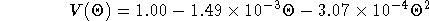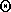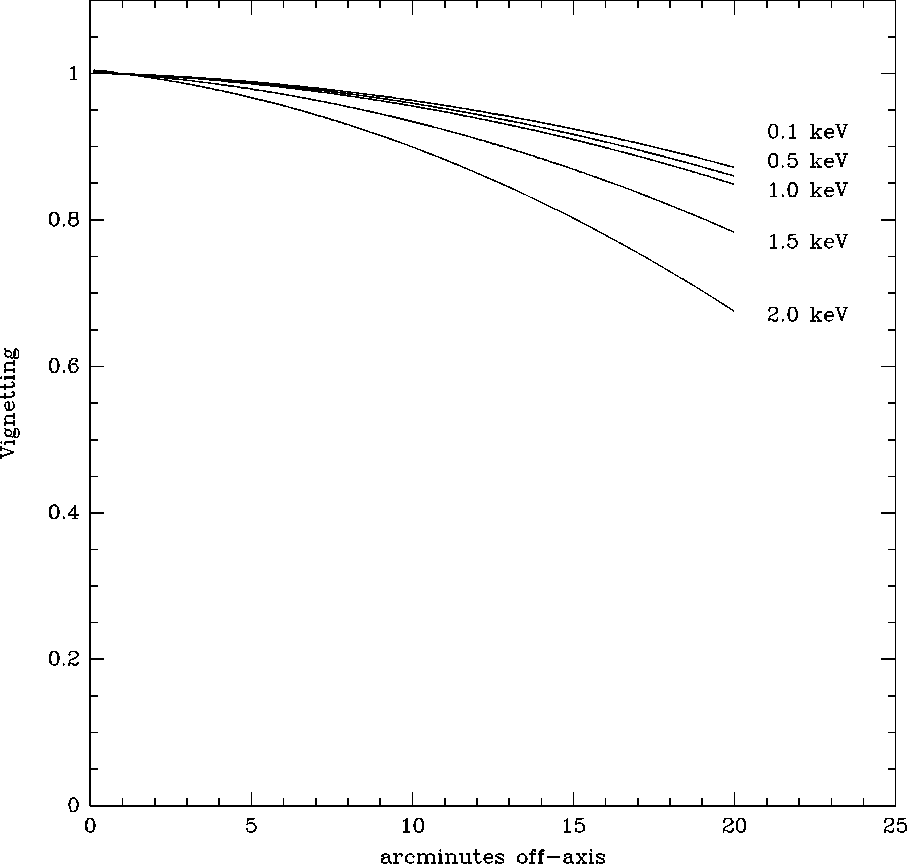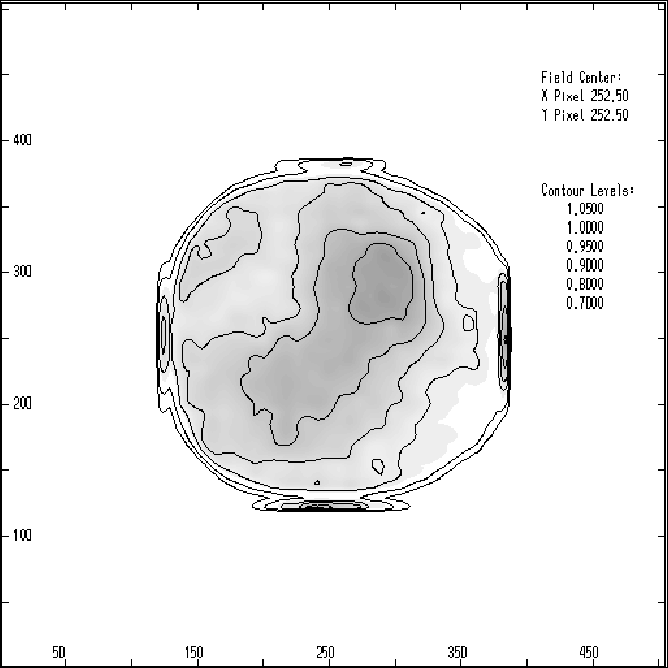Next: Long-term stability Up: Effective Area Previous: On-axis Effective Area

### Off-axis Effective Area

The XRT effective area varies smoothly with off-axis angle and energy. Figure 5.12 shows the XRT vignetting function (normalized to unity on-axis) for a range of photon energies. Vignetting is less than a 10% effect within 10' at all energies of interest. The vignetting function at 1.0 keV is well fit with the following parametric representation:whereis in units of arc minutes.

The spatial uniformity of the HRI quantum efficiency   was measured six months before launch in a series of flat field tests at three energies: 0.28, 0.93, and 1.49 keV. The quantum efficiency of the HRI varies by less than 10% over the detector and is nearly energy independent. Figure 5.13 is a representative contour plot of the spatial variation in the quantum efficiency of the HRI.Figure 5.12: The vignetting function of the XRT at 5 photon energies in the HRI field of view. The vignetting function is normalized to unity on-axis.Figure 5.13: Contours of the HRI QE map used by the on-line processing (SASS). The image has been blocked by a factor of 16 yielding 8'' pixels. The QE map shown in this figure was generated by combining ground based flat field measurements at C(0.28 keV), Cu(0.93 keV), and Al(1.49 keV) in the correct proportions for a power law spectrum with an energy index of -1. The composite image was then smoothed with a 32'' gaussian producing statistical errors of 1%. The QE is normalized to unity on-axis and the contours are at 0.7, 0.8, 0.9, 0.95, 1.0, 1.05 and decrease outward. Note that the QE of the HRI varies by less than 10% over most of the field of view.

If you have problems/suggestions please send mail to rosat_svc@mpe-garching.mpg.de